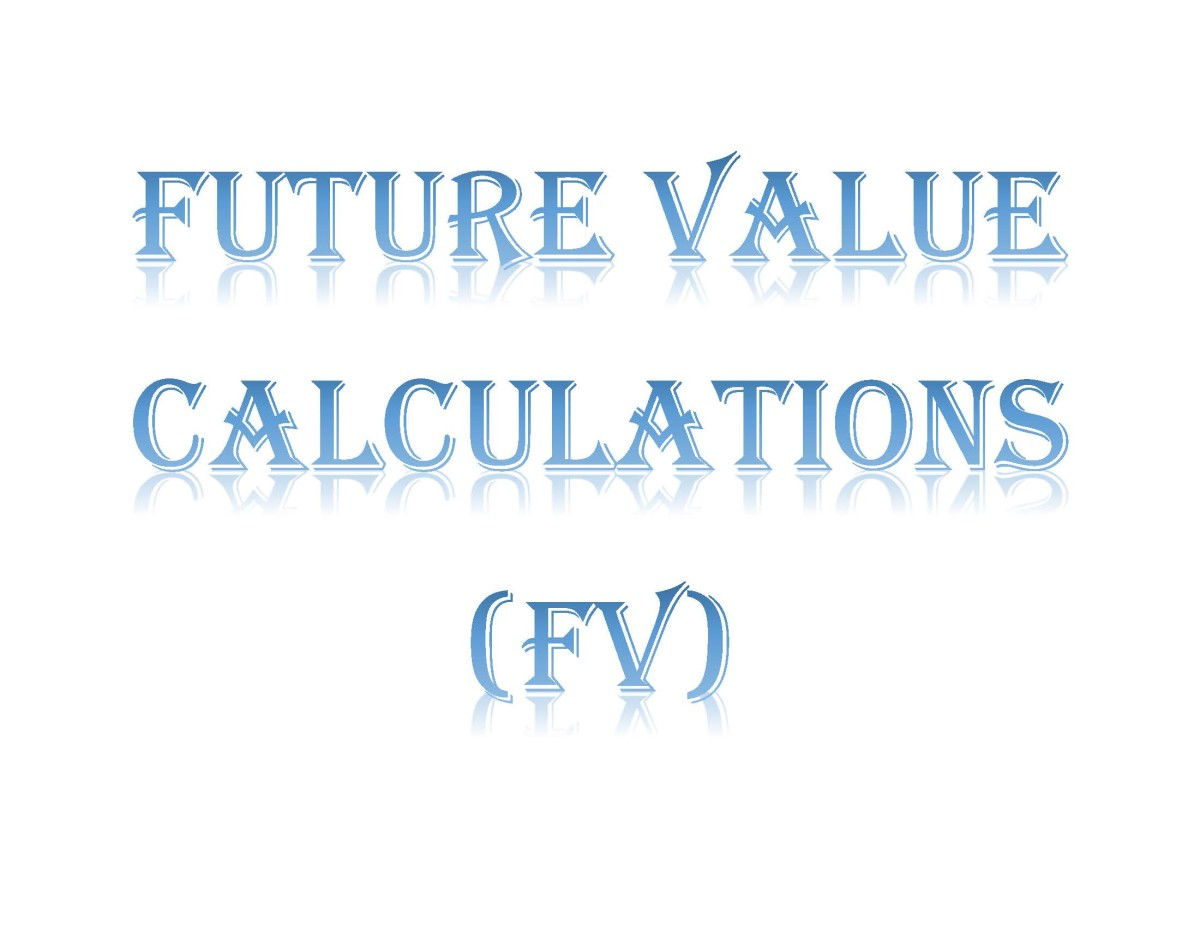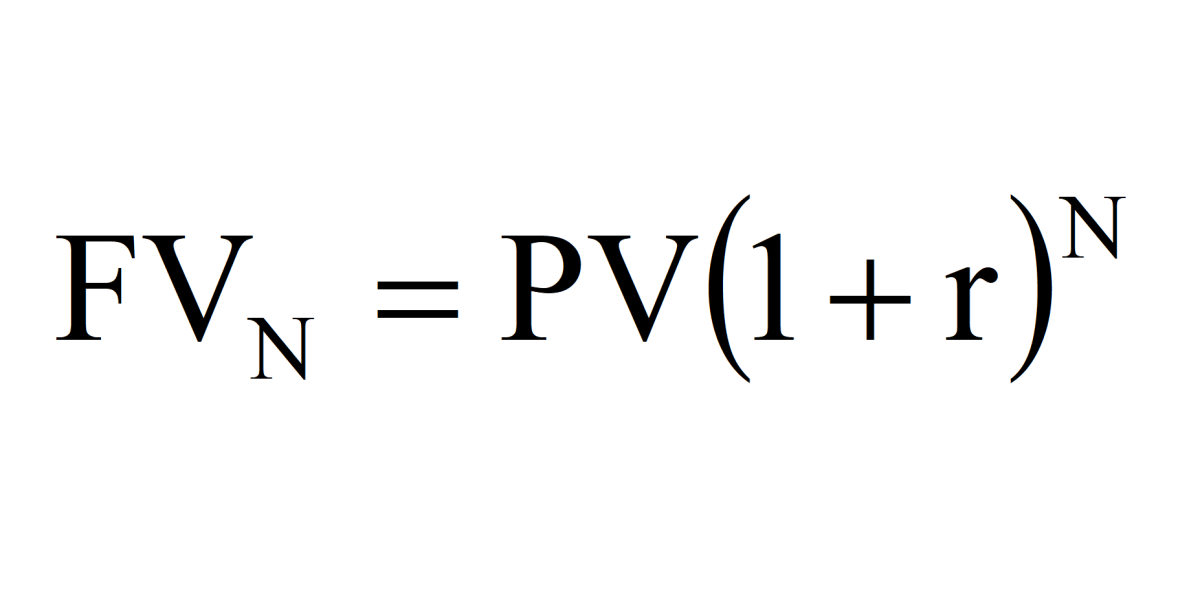Updated:
Original:

# How to Find Future Values (TVM Calculations)

Joshua is a graduate student at the USF. He has interests in business technology, analytics, finance, and lean six sigma.Being able to calculate the time value of money is a valuable skill for business and personal finance. Created by Joshua Crowder

Finding the future value of a financial asset is a requirement for any student pursuing a career in management or the financial sector. These time value of money problems are very simple to solve with multiple methods available that can be used to solve the problem.

The future value of an asset is the measurement of the nominal future sum of money that a sum of money is worth at an interest rate and at a specified future date. Simply put, it is the value of an asset over a specified period. In this tutorial several ways to calculate the future value of a financial instrument will be covered.

## Creating a Timeline

A great start to completing any time value of money (TVM) calculation is to create a time line. Below is a number line for the following example that will be calculated for the future value. A visual of the period of compounding is a great reference and recommended for all types of (TVM) problems.

The \$500 (representing present value) on the time-line is money that you have in a bank account today. We want to find the future value of the money you would have after 3 years’ time passed. The zero on the time line represents today followed by each year the investment is made. This account will compound interest at 5% each year.

The number line is great to use as a visual and or it can be used to solve the problem using the step-by-step approach.

## Step-by-Step Approach for Compounding Interest

The step-by step approach is very straight forward. The time line drawn previously can be utilized for this method. The time line is modified as the problem progresses. Essentially, you will be completing the problem in steps as the title suggests.

### Step 1

To find the percentage of interest earned after 1 year for the example above multiply .08 x \$500 = \$40 interest. Forty dollars is the interest earned after one year.

### Step 2

The \$40 interest is then added to the \$500 that was initially invested.

### Step 3

For the next year multiply .08 x \$540 = \$43.20 interest.

### Step 4

Next, add the \$43.20 interest from year two to \$540 and year 2 will accumulate to \$583.20.

### Step 5

At the start of year 3, the money is compounded again where .08 x \$583.20 = \$46.66 interest.

### Step 6

After adding this interest to the previous balance of \$583.20 the future value at year 3 is \$629.86.

The step-by-step approach is the longest method but good for showing how the interest compounds year by year. Realistically you will not be using this method unless other tools are not available to you. The following methods will find the solution in a more timely manner.Future value time value of money formula. The future value formula is the present value times the accumulation function. Created by Joshua Crowder

## The Future Value (FV) Formula Approach

The calculate the future value using the formula approach you must simply plug in needed variables to solve for future value or FVn in the formula FVn=PV(1+r)n. The present value (PV) in this equation is the initial negative cash flow that was invested for a period to receive future interest earnings. The “n” in the equation is the number of compounding periods that the investment will incur. The “n notation” to the right of FV means the future value is the future value of whatever n number of compound periods is. The equation for this problem is shown and solved below by plugging in all variables:

FVn=PV(1+r)n

=500(1+.08)3

=500(1.08)3

=500(1.2597)

=629.856

=629.86

Take notice that 8 percent must be entered to the formula in decimal form and the 500 must be entered into the formula as a positive number. The formula method is relatively quick compared to the step-by-step method.

## Using an HP 10BII+ Calculator

Finding the future value of a TVM problem with a calculator is an efficient method. Using an HP 10BII+ is not much different from using other financial calculators. The first step on the HP 10BII+ is to press the orange shift key that points down and C ALL to clear out the TVM register. For 3 years, 3 would have to be typed followed by the N button. This will enter 3 as the number of years in the TVM register. Next, 8 (non-decimal) is typed followed by the I/Y button to enter the interest rate. The initial investment value -500 is typed followed by PV. The present value is entered as a negative because an investment is a negative cash flow (amount that was invested out of someone's pocket). Zero should be entered for the payment value. Each TVM register value is listed below:

N=3

I/Y=8

PV= -500

PMT=0

To find the present value just press the FV key. The value should be 629.856.

## Using a TI BAII Plus Calculator

Using a TI BAII Plus calculator is not much different from using an HP 10BII+. First, make sure that the TVM register is clear by pressing 2cd → CLR WORK, then press 2cd → CLR TVM. Now values can be plugged into the TVM register. So, for 3 years, 3 would have to be typed followed by N. This will enter 3 as the number of years in the TVM register. Next, 8 (non-decimal) is typed followed by I/Y to enter the interest rate. The initial investment value -500 is typed followed by PV. The present value is entered as a negative because an investment is a negative cash flow (amount that was invested out of someone's pocket). Zero should be entered for the payment value. Each TVM register value is listed below:

N=3

I/Y=8

PV= -500

PMT=0

Now that all the required variables are added to the TVM register, press CPT → FV. The value 629.856 should display if your calculator is set to display at least 3 decimal places.

## Using Microsoft Excel

Using an Excel spreadsheet to find the future value of the above example is a very popular option.
When you get into Excel all you need to do is type =FV(.08,3,0,500,1). All Excel functions have a very specific format. Within the parenthesis of this function the first value typed is the rate which must be typed in decimal form. Next, the number of periods that asset will be compounding. After that, the present value or the initial investment in added. Note that in this calculation the present value in added as a positive number. And finally, the last value dictates whether the compounding will occur at the beginning of the period or at the end. The number 1 compounds at the beginning and 0 will compounds at the end.

• Future Value. (2018, June 25). Retrieved July 02, 2018, from https://en.wikipedia.org/wiki/Future_value
• Brigham, E. F., & Ehrhardt, M. C. (2017). Financial management: Theory and practice. Boston (MA: Cengage Learning.

This content is accurate and true to the best of the author’s knowledge and is not meant to substitute for formal and individualized advice from a qualified professional.